1. What is ( 999 − 99 + 9 ) ÷ 9 ?

A:
B:
C:
D:
E:

2. How many minutes are there in 1/12 of a day?

A:
B:
C:
D:
E:

3. In my row in the theatre the seats are numbered consecutively from T1 to T50. I am sitting in seat T17 and you are sitting in seat T39.

How many seats are there between us?

A:
B:
C:
D:
E:

4. The number 987 654 321 is multiplied by 9.

How many times does the digit 8 occur in the result?

A:
B:
C:
D:
E:

5. What is the difference between the smallest 4-digit number and the largest 3-digit number ?

A:
B:
C:
D:
E:

The diagram shows a square divided into strips of equal width.

Three strips are black and two are grey.

What fraction of the perimeter of the square is grey?

A: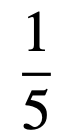B: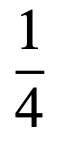C: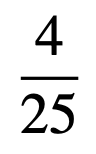D: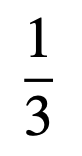E: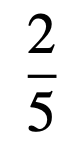7. What is 2014 − 4102?

8. How many prime numbers are there in the list

1, 12, 123, 1234, 12 345, 123 456 ?

A:B:C:D:E:9. Triangles XYZ and PQR are drawn on a square grid.

What fraction of the area of triangle XY Z is the area of triangle PQR?

A: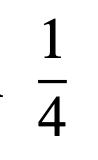B: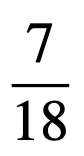C: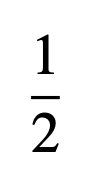D: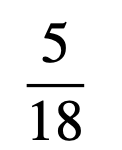E: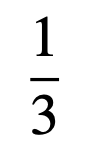10. An equilateral triangle is surrounded by three squares, as shown.

What is the value of x?

A:B:C:D:E:11. The first two terms of a sequence are 1 and 2.

Each of the following terms in the sequence is the sum of all the terms which come before it in the sequence.

Which of these is not a term in the sequence?

A:B:C:D:E:12. In this subtraction, P, Q, R, S and T represent single digits.

What is the value of P + Q + R + S + T ?

A:B:C:D:E:13. A rectangle is split into triangles by drawing in its diagonals.

What is the ratio of the area of triangle P to the area of triangle Q?

A:B:C:D:E:14. Which of these is equal to one million millimetres?

A:B:C:D:E:15. The diagram shows a rectangular envelope made by folding (and gluing) a single sheet of paper.

What could the original unfolded sheet of paper look like?

(The dashed lines are the fold lines.)

A: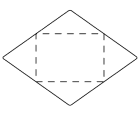B: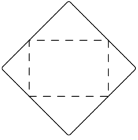C: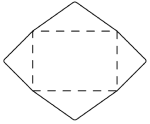D: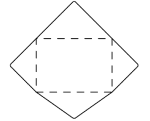E: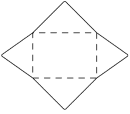Back to the top